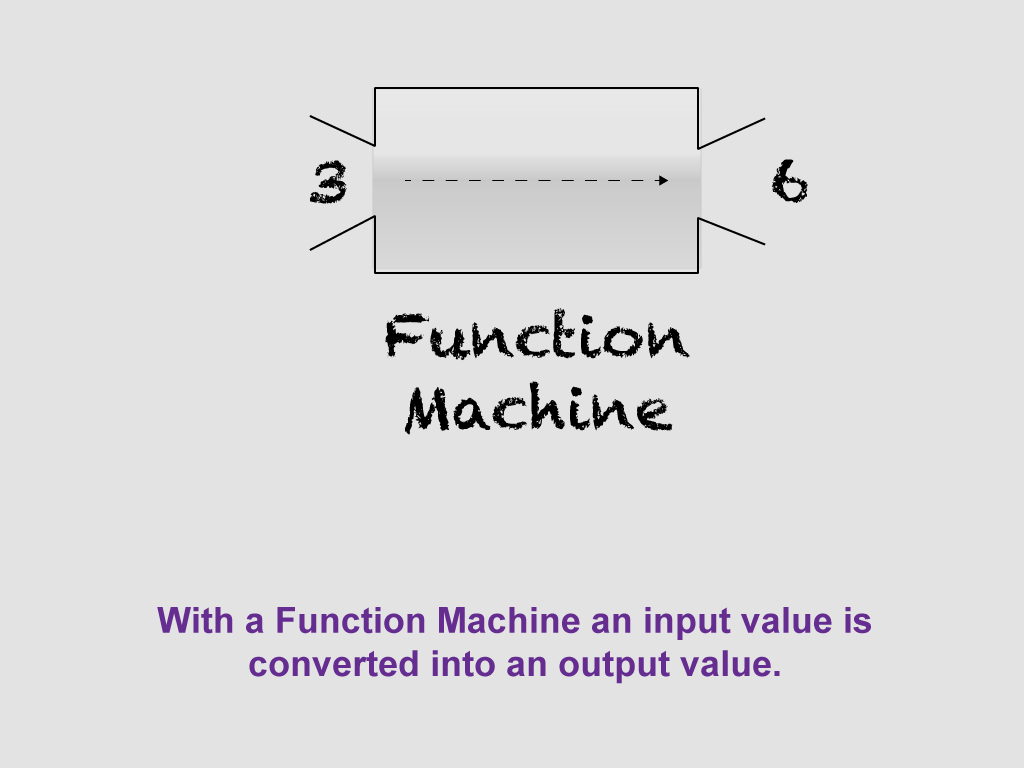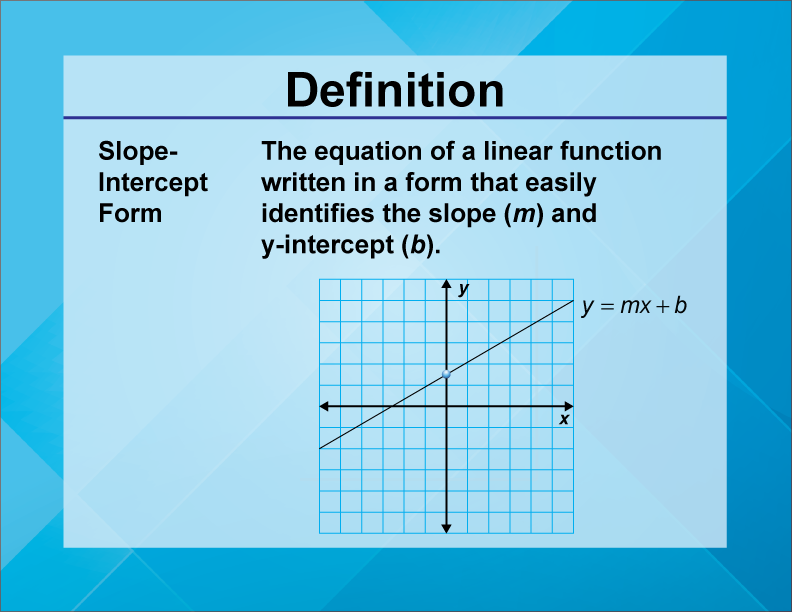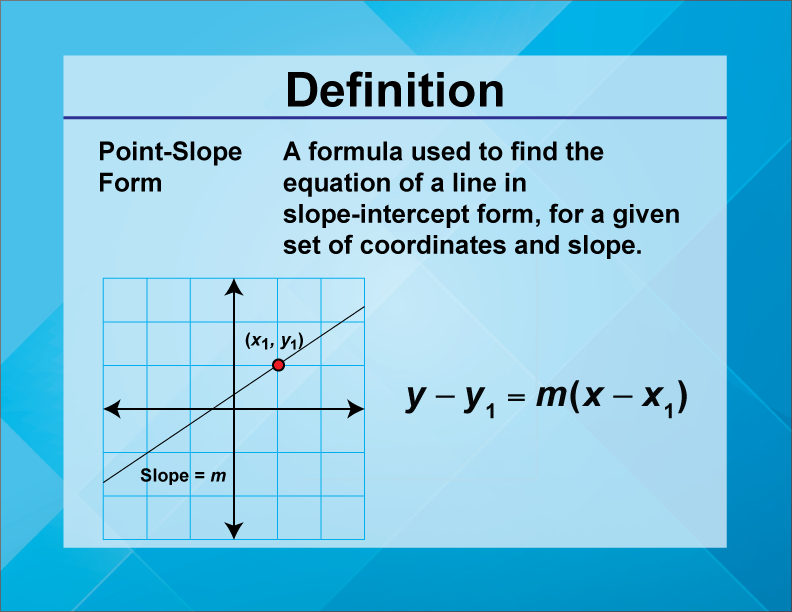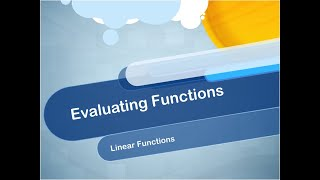# Worksheet: Evaluating Linear Functions, Set 7

This is part of a collection of math worksheets on the topic of evaluating linear functions.

### To see the complete collection of worksheets on this topic, click on this link.

The following section provides a review of linear functions. You may want to read through it and watch the companion video before completing the worksheet.

## Brief Review of Functions

Before diving into the topic of linear functions, let's review the following topics, which pertain to all functions.:

• Definition of a function

• Independent variable

• Dependent variable

• Domain

• Range

• Different representations of functions

What Is a Function? A function is a one-to-one mapping of input values (the independent variable) to output values (the dependent variable). Click on this link to see a quick tutorial on what a function is. This slide show goes over the following key points:

• For every input value (x), there is a unique output value, f(x).

• Functions can be represented as equations, tables, and graphs.

• A function machine is a useful visual representation of the input/output nature of functions.Dependent/Independent Variables. When one variable depends on another, then it is the dependent variable. For example, the faster your speed, the farther you travel. Suppose that speed is represented by the variable s and the distance traveled is represented by the variable d.

Here's how to describe the relationship between s and d:

The faster the speed, the more distance traveled.

Distance is dependent on speed.

Distance is a function of speed.

### d = f(s)

When studying functions, make sure you are comfortable telling the difference between the independent variable and dependent variable. Get comfortable using function notation. To learn more about function notation, click on this link.

Domain and Range. A function shows the relationship between two variables, the independent variable and the dependent variable. The domain is the allowed values for the independent variable. The range is the allowed values for the dependent variable. The domain and range influence what the graph of the function looks like.

For a detailed review of what domain and range are, click on this link to learn more. You’ll see definitions of the terms domain and range, as well as examples of how to find the domain and range for given functions.

Multiple Representations of Functions. We mentioned previously that functions can be represented in different ways. In fact, any function can be represented by an equation, usually f(x) equal to some expression; a table; or a graph. For a detailed review of multiple representations of functions, click on this link, to see a slide show that includes examples of these multiple representations.

## What Are Linear Functions?

The most important form of a linear function is the slope-intercept form.Given the slope, m, and the y-intercept, b, for a linear function, you can easily construct the equation and graph of the linear function. To see examples of graphing linear functions in slope-intercept form, click on this link. This slide show also includes a video tutorial.

## Point-Slope Form

Another method of finding the slope-intercept form involves using the point-slope form. In this case you are given the slope, m, of the line and one set of coordinates, (x, y), on the line. This is what the point-slope form looks like.To see examples of deriving the linear function using the  in point-slope form, click on this link. This slide show tutorial walks you through the process and provides several worked-out examples.

## Evaluating Linear Functions

In this video learn about linear functions and how to evaluate them for different values of x.The video was uploaded on 10/7/2022.

You can view the video here.

The video lasts for 2 minutes and 26 seconds.